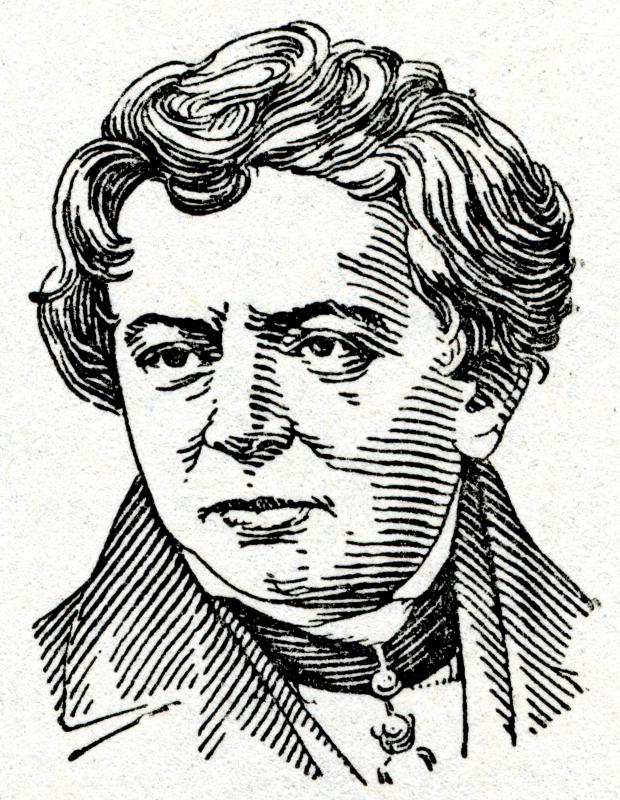# What is an Electrical Unit?

Alex Paul
Alex Paul

An electrical unit is any unit of measurement that is used to describe a property found in electric circuits. Examples of some of the most common types of electrical unit include a coulomb, which is used for measuring charge; an ampere, which is used for measuring electrical current; and a volt, which is used to measure voltage. Electric units provide an absolute measurement of the state of a particular circuit at any one time, which is essential for building and maintaining electrical circuits.Electrical resistance was discovered by German physicist Georg Ohm in the 19th century and has since been measured in ohms.

The unit of voltage — the volt — is probably one of the most important electrical units. It is also sometimes known as the unit of electromotive force. This second name provides a clue as to what voltage actually is — a force that acts on electrons in a circuit and pushes them in a certain direction. The volt is also the electrical unit for potential difference which is a similar quantity.Individuals pay per kilowatt hour for the electricity they use in their homes.

Current is the flow of electrons around an electrical circuit. The electrical unit of current is the ampere, which describes the amount of charge flowing per second. For this reason the ampere can also be described as coulombs per second. At a basic level the current is a measurement of how many electrons are passing a certain point every second. This is due to the fact that each electron has a specific charge.

Aside from voltage and current the third basic electrical property is resistance and this has the unit of ohms. Electrical resistance describes the strength of opposition to the flow of electrons round a particular circuit. Although specially made resistors are used to increase the resistance in a circuit and hence reduce current any component has an inherent resistance. Even wires have a small but real resistance which increases with temperature.

Other electrical units include the watt, which is a measure of electrical power, and farad, which is a measure of capacitance. The joule is a standard unit in physics for energy although it can also be applied to electrical energy flowing round a circuit. A joule, however, is a relatively small unit, which is why kilowatt-hours — a more practical measurement of energy — is commonly used in many situations.

The coulomb is considered to be the standard electrical unit as it’s a measurement of charge. It can also be considered as the amount of electricity transferred through a circuit in one second by a certain current. Equations linking these standard properties of an electrical circuit allow for detailed predictions of how electricity will behave in a certain situation.

## You might also Like